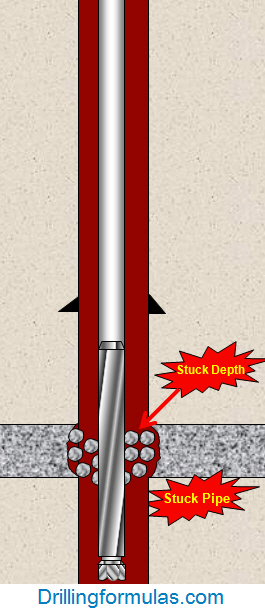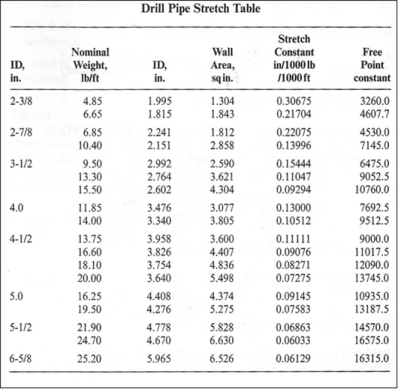## Determine Stuck Pipe Depth Based on Real Example

This is the real example of how to determine stuck depth using the stuck depth calculation.

Well Information:

• TD = 8,900’MD/5,600’ TVD
• Bit Size = 8.5”
• Drilling fluid = water based mud (PHPA system)
• Formation: sand shale sequence
• BHA: 7” mud motor + 9 stands of 5” HWDP S-135, 4-1/2” IF connection
• Drillstring: 5” DP S135, 4-1/2” IF connection
• Neutral weight @ 6,850’MD = 175 Klb

While tripping out of hole to 6,850’MD, observe over pull 40 Klb over pick up weight. Stop tripping out and attempt to go down no issue. Attempt to rotate, pipe is able to rotate at 30K ft-lb. Attempt to circulate, observe restricted flow.Figure 1 – Stuck While Pulling Out Continue reading

## Determine how many feet of free pipe without free pipe constant table

You may sometimes find the table for Free Point Constant for your stuck pipe calculation so you use the formula from the previous post to determine Free Point Constant and apply it into free pipe formula to determine where the depth of stuck pipe is.Stuck Pipe Calculation – Stuck Depth

The steps of calculation are as follows:

1 ) Determine the free point constant (FPC) by this following formula:

FPC = As x 2500

where: As = pipe wall cross sectional area, sq in.

As = (OD2-ID2) x 0.7854

OD and ID are inch unit

2) Determine depth of stuck pipe by this following formula:

Depth of stuck pipe = (Pipe stretch in inch x free point constant (FPC)) ÷ Pull force in thousands of pounds

Please see the example below to demonstrate you how to apply those 2 formulas above into real drilling operation.

Example#2: Determine the free point constant (FPC) and the depth the pipe is stuck using the following information:

3-1/2” tubing # 9.5 lb/ft

3-1/2” tubing ID = 2.992 inch

20 inch of stretch with 25 Klb of pulling force

a) Determine free point constant (FPC):

FPC = (3.52 – 2.9922) x 0.7854 x 2500

FPC = 6475.5

b) Determine the depth of stuck pipe:

Feet of free pipe (ft) = (20 inch x 6475.5) ÷ 25

** 25 is the kilo-force to pull to 20 inch **

Feet of free pipe (ft) = 5,180 ft

Please find the Excel sheet used for calculating depth of stuck pipe.

## Determine free point constant (FPC)

Referring to the previous post, it is difficult to find drill pipe stretch table or there is no data available for you when you are really need it. This post will show you how to calculate free point constant (FPC) based on cross section of drill pipe, tubing or any cylindrical objects.

The free point constant (FPC) formula for any type of steel drill pipe is listed below:

FPC = As x 2500

where: As = pipe wall cross sectional area, sq in.

As = (OD2-ID2) x 0.7854

This method requires outside diameter and inside diameter data.

Example#1: Determine free point constant (FPC) of 4 in. drill pipe 14.0 lb/ft, inside diameter of drill pipe = 3.34 inch.

FPC = (42 – 3.342) x 0.7854 x 2500

FPC = 9512

Please find the Excel sheet to calculate free point constant.

## Determine how many feet of free pipe

Stuck pipe is one of serious situations that sometimes happen on the rig. People put a lot of effort to free stuck pipe; however, when they reach the point that they can not free the pipe anymore, they may need to know where the stuck point is in order to plan to cut or back off drill pipe. This post will demonstrate you how to determine free point constant and calculate where a stuck point is.

Firstly, we need to know the free point constant which can be found by this following table.Secondly, determine the depth where drill string gets stuck  by  the following formula.

Depth of stuck pipe in feet = (Pipe stretch in inch x free point constant (FPC)) ÷ Pull force in thousands of pounds

Example: Determine where the stuck pipe depth is by given information: 3-1/2 in. 9.5 ppf drill pipe, 25 inch of stretch with 30,000 lb of pull force

From drill pipe stretch table, the free point constant is 6475 for 3-1/2 in. drill pipe 9.5 ppf.

Feet of free pipe = (25 x 6475) ÷30

Feet of free pipe = 5396 ft

This mean there are 5396 ft of free drill pipe so when you know this figure, you can plan your wireline work for backing off drill pipe, colliding drill pipe, etc.

Please find the Excel sheet to calculate free pipe.

Ref books:

Lapeyrouse, N.J., 2002. Formulas and calculations for drilling, production and workover, Boston: Gulf Professional publishing.

Bourgoyne, A.J.T., Chenevert , M.E. & Millheim, K.K., 1986. SPE Textbook Series, Volume 2: Applied Drilling Engineering, Society of Petroleum Engineers.

Mitchell, R.F., Miska, S. & Aadny, B.S., 2011. Fundamentals of drilling engineering, Richardson, TX: Society of Petroleum Engineers.